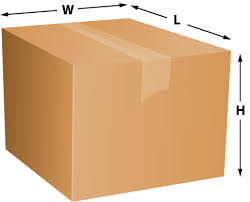How Billable Weight of Your Package is Calculated

With WizMe and other carriers, the billable weight of a package is based on the greater of actual weight or cubic weight.

Shipping costs were once calculated on the basis of gross weight in kilograms. But by charging only by weight, lightweight, low density packages became unprofitable for freight carriers due to the amount of transportation space taken by those packages.

Therefore the concept of dimensional / volumetric weight or cubic weight was introduced by the transportation industry worldwide as a uniform means of establishing a minimum charge for the cubic space a package occupies. Cubic weight, is a pricing technique which uses an estimated weight that is calculated by:

• Measuring the package length, width and height in centimetres
• Multiplying length x width x height
• Divide that result by 1,000 to calculate the package volume in litres
• Then divide volume by 4 to calculate Cubic Weight.

EXAMPLE:

Take a package with these dimensions:• Length = 40 cm
• Width = 30 cm
• Height = 20 cm
• Actual weight = 4kg

Volume = 40 x 30 x 20 divided by 1,000 = 24 Litres

Cubic Weight = 24 divided by 4 = 6kg cubic

Therefore the Billable Weight for shipping would be assessed on the basis of 6kg’s.

Use Rate Calculator at QUICK QUOTE here.